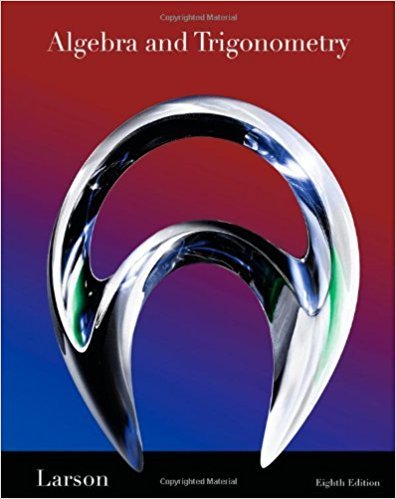×
×

# Solutions for Chapter 2.6: Combinations of Functions: Composite Functions## Full solutions for Algebra and Trigonometry | 8th Edition

ISBN: 9781439048474Solutions for Chapter 2.6: Combinations of Functions: Composite Functions

Solutions for Chapter 2.6
4 5 0 305 Reviews
27
3
##### ISBN: 9781439048474

This expansive textbook survival guide covers the following chapters and their solutions. Since 86 problems in chapter 2.6: Combinations of Functions: Composite Functions have been answered, more than 47457 students have viewed full step-by-step solutions from this chapter. Algebra and Trigonometry was written by and is associated to the ISBN: 9781439048474. This textbook survival guide was created for the textbook: Algebra and Trigonometry, edition: 8. Chapter 2.6: Combinations of Functions: Composite Functions includes 86 full step-by-step solutions.

Key Math Terms and definitions covered in this textbook
• Complete solution x = x p + Xn to Ax = b.

(Particular x p) + (x n in nullspace).

• Cramer's Rule for Ax = b.

B j has b replacing column j of A; x j = det B j I det A

• Full column rank r = n.

Independent columns, N(A) = {O}, no free variables.

• Gauss-Jordan method.

Invert A by row operations on [A I] to reach [I A-I].

• Graph G.

Set of n nodes connected pairwise by m edges. A complete graph has all n(n - 1)/2 edges between nodes. A tree has only n - 1 edges and no closed loops.

• Hermitian matrix A H = AT = A.

Complex analog a j i = aU of a symmetric matrix.

• Independent vectors VI, .. " vk.

No combination cl VI + ... + qVk = zero vector unless all ci = O. If the v's are the columns of A, the only solution to Ax = 0 is x = o.

• Left nullspace N (AT).

Nullspace of AT = "left nullspace" of A because y T A = OT.

• Multiplication Ax

= Xl (column 1) + ... + xn(column n) = combination of columns.

• Normal matrix.

If N NT = NT N, then N has orthonormal (complex) eigenvectors.

• Pivot columns of A.

Columns that contain pivots after row reduction. These are not combinations of earlier columns. The pivot columns are a basis for the column space.

• Row space C (AT) = all combinations of rows of A.

Column vectors by convention.

• Schur complement S, D - C A -} B.

Appears in block elimination on [~ g ].

• Singular matrix A.

A square matrix that has no inverse: det(A) = o.

• Singular Value Decomposition

(SVD) A = U:E VT = (orthogonal) ( diag)( orthogonal) First r columns of U and V are orthonormal bases of C (A) and C (AT), AVi = O'iUi with singular value O'i > O. Last columns are orthonormal bases of nullspaces.

• Skew-symmetric matrix K.

The transpose is -K, since Kij = -Kji. Eigenvalues are pure imaginary, eigenvectors are orthogonal, eKt is an orthogonal matrix.

• Spanning set.

Combinations of VI, ... ,Vm fill the space. The columns of A span C (A)!

• Stiffness matrix

If x gives the movements of the nodes, K x gives the internal forces. K = ATe A where C has spring constants from Hooke's Law and Ax = stretching.

• Transpose matrix AT.

Entries AL = Ajj. AT is n by In, AT A is square, symmetric, positive semidefinite. The transposes of AB and A-I are BT AT and (AT)-I.